F90中，允许把整个数组作为一个操作数进行操作，也允许在赋值语句中对整个数组进行赋值，就像对一个简单变量的操作和赋值一样。针对数组的特性，也有专门的运算方法和内在函数。

5.2.1 赋值

a)    赋值方式

F90数组赋值语句的形式为：V=eV代表数组名或数组片段，e为数组表达式，它们必须有相同的形状（即维数相同，每维长度相同但上下界可不同）。当V大小为0或为长度为0的字符型时，则没有值赋给V。当e为标量时，则把e处理成与V相同的形状，V的每个元素均等于标量e的值。数组表达式e中允许使用+-*/**等内部算术操作符。

F90允许赋值语句的表达式内只有标量，如A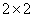数组，则A=3表示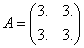。例如，A(1:15)=2.0，则A(1)A(15)的每个元素都被赋值为2.0。因此，数组赋值语句可以对整个数组一次赋值完毕，不必用DO构造对每一个元素逐一赋值，如对F77中的初始化置零DO循环：

do i=1,10

do j=1,10

A(i,j)=0.

end do

end do

A(1:20)=A(20:1:-1)                ! A数组元素值倒序重排

A=(/1,2,3,4,5,6/)                 ! 斜杠与括号间不能有空格

C2=(/B(I,1:5),B(I:J,7:9)/)        ! 数组表示

C3=(/(I,I=1,4)/)                  ! DO循环

C4=(/4,A(1:5),(I,I=1,4),7/)       ! 混合表示

1) 方括号代替括号和斜线，例如下面两个数组构造器是等价的：

C=(/4,8,7,6/)

C=[4,8,7,6]

2) 冒号三元下标(代替隐DO循环)来指定值的范围和步长，例如下面两个数组构造器是等价的：

D=(/1:5:2/)               ! 三元下标格式

D=(/(I,I=1,5,2)/)         ! DO循环格式

b)    数组的存储顺序c)    DO循环的差异

F90的赋值语句考虑到了在并行机上计算的功能，即使不是用的并行计算机，在形式上也是按并行化处理的。这与F77中的串行赋值是不同的，由此造成了数组的F90赋值结果与采用DO循环方式进行赋值的差异。

a(1:9)=a(0:8)

a的所有元素是并行处理的，结果是(/0,0,1,2,3,4,5,6,7,8/)

|a(0)|a(1)|a(2)|a(3)|a(4)|a(5)|a(6)|a(7)|a(8)|a(9)|

|a(0)|a(1)|a(2)|a(3)|a(4)|a(5)|a(6)|a(7)|a(8)|a(9)|

DO i=1,9

a(i)=a(i-1)

END DO

A的元素是逐一处理的，结果是(/0,0,0,0,0,0,0,0,0/)

|a(0)|a(1)|a(2)|a(3)|a(4)|a(5)|a(6)|a(7)|a(8)|a(9)|

INTEGER :: a(0:9)=(/0,1,2,3,4,5,6,7,8,9/), b(0:9)

b=a

DO i=1,9

a(i)=b(i-1)

END DO

d)    RESHAPE语句

REAL F(5,3,8),C(8,3,5)

AR1=RESHAPE((/1,2,3,4,5,6/),(/2,5/),(/0,0/),(/2,1/))

! AR1的取值为     [1 2 3 4 5]

!                 [6 0 0 0 0]

C=RESHAPE(F,(/8,3,5/),ORDER=(/3,2,1/))   ! Fortran数组序化为C数组序

END

B=RESHAPE((/1,2,3,4,5,6/),(/2,3/))       ! 赋值给形状为(2,3)的数组

C=(/O,RESHAPE(B,(/6/)),7/)               ! 赋值给向量C之前先把B转换成向量

e)    WHERE构造

F90中提供了屏蔽数组赋值语句，它实质上是一种带条件的数组赋值语句，也就是说它只对某些符合条件的数组元素赋值。屏蔽数组赋值语句，为WHERE语句和WHERE构造，其形式非常类似于逻辑IF语句和IF构造。

WHERE语句的一般形式为：WHERE(屏蔽表达式) 赋值语句

WHERE构造的一般形式为：

[构造名:] WHERE(屏蔽表达式1)

[]

[ELSEWHERE(屏蔽表达式2) [构造名]

[]]

[ELSEWHERE [构造名]

[]]

END WHERE [构造名]

B=.FALSE.

WHERE(ABS(A)>1.0) B.TRUE.

WHERE(ABS(A)>1.0) A1./A

B=.FALSE.

WHERE(ABS(A)>1.0)

B.TRUE.

A1./A

END WHERE

WHERE(ABS(A)>1.0)

B.TRUE.

A1./A

ELSEWHERE

B=.FALSE.

END WHERE

DIMENSION A(5),B(5),C(5)

DATA A/0,1,1,1,0/

DATA B/10,11,12,13,14/

C=-1

WHERE(A.NE.0) C=B/A

f)    FORALL屏蔽赋值

FORALLF95的新增功能。它是数组屏蔽赋值（WHERE语句和构造）功能的一对一元素的推广，其方式有FORALL语句和FORALL构造。

FORALL语句的一般形式为：FORALL(循环三元下标[,循环三元下标][,屏蔽表达式]) 赋值语句

FORALL构造的一般形式为：

[构造名:] FORALL(循环三元下标[,循环三元下标][,屏蔽表达式])

[]

END FORALL [构造名]

FORALL(i=0:7,j=0:7) mat(i,j)=8*i+j

FORALL(i=0:7,j=0:7)

mat(i,j)=8*i+j

END FORALL

DO i=0,7

DO j=0,7

mat(i,j)=8*i+j

END DO

END DO

` `

WHERE(a>0.0)  a=SQRT(a)

FORALL(i=0:n,a(i)>0.0) a(i)=SQRT(a(i))

` `

FORALL(I=1:N,J=1:N,A(I,J).NE.0.) B(I,J)=1./A(I,J)

FORALL (I=1:N,J=1:N)

IF(A(I,J).NE.0.) B(I,J)=1./A(I,J)

END FORALL

` `

FORALL(i=1:7) num(i)=num(i-1)

FORALL(i=1:7)

num(i)=num(i-1)

END FORALL

a(1:7)=a(0:6)

DO i=1,7

num(i)=num(i-1)

END DO

`[e_521_01.f90]`

5.2.2 运算

a)    基本运算

even=(/(2*i,i=0,49)/); odd=(/(2*i+1,i=0,49)/)

plu=even+odd

min=even-odd

tim=even*odd

div=even/odd

squ=even**2

INTEGER D(5)

DATA PI/3.14159265/

A=(/(REAL(I)*PI/180.,I=1,5)/)

B=COS(A); C=SQRT(A); D=CEILING(A*180.)

A=20.; B=5.; A=A-B(2,1:5)

b)    数组与数组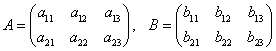,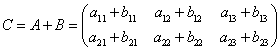c)    数组与标量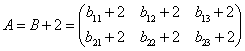,

d)    数组内在函数

F90中增加了许多新的数组专用内在函数，使得数组的运算更加灵活方便。下面是一部分新增的数组内在函数。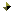矩阵乘积函数MATMUL(A,B)向量点乘函数DOT_PRODUCT(A,B)数组归约函数：包括SUMPR0DUCTMAXVALMINVALCOUNTANYALL函数。数组查询函数：包括SIZESHAPEALLOCATEDLBOUNDUBOUND函数。数组的构造函数：包括MERGEPACKUNPACKSPREAD函数。

SPREAD(A(2,2:4),1,3)SPREAD(A(1:3,1),2,3)数组重构形函数：RESHAPE(SOURCE,SHAPE[,PAD][,ORDER])数组运算函数：包括TRANSPOSEEOSHIFTCSHIFT三个函数。

EOSHIFT(B,SHIFT=1,BOUNDARY='*',DIM=2)EOSHIFT(B,SHIFT=-1,DIM=1)EOSHIFT(B,SHIFT=(/1,-1,0/),BOUNDARY=(/'*','?','/'/),DIM=2)数组定位函数：有MAXLOCMINLOC两个函数。

INTEGER, DIMENSION(0:6),PARAMETER :: A=(/3,7,0,-2,3,6,-1/)

F90数组运算内在函数表

e)    数组的输入输出一维数组:

READ *, (a(i), i=1,10)            ← 可以1行输入10个值，也可输入1个值(DO循环)

READ *, a                         ← 同上           (数组名)

DO i=1,10

END DO

PRINT '(10I5)',(a(i),i=1,10)      1行打印10个值 (DO循环)

PRINT '(10I5)',a                  ← 同上           (数组名)

PRINT '(5I5)',a(1:5)              1行打印5个值  (数组片段)

DO i=1,10

PRINT '(I5)',a(i)              1行打印1个值，共打10

END DO二维数组:

5.2.3 数组的动态分配

a)    可分配数组

b)    ALLOCATE语句

ALLOCATE语句动态创建可分配数组，使内存和对象相联系。分配的对象可以被命名为任何有ALLOCATABLE属性的变量。它的一般形式为：

ALLOCATE(数组名[维界符][,数组名[(维界符[,维界符...])] ] ...[,STAT=状态值])

ALLOCATABLE A,B

ALLOCATE(A(-2:40),B(3,3,3))

INTEGER ERR_MESSAGE

ALLOCATE(A(10:25),B(SIZE(A)),STAT=ERR_MESSAGE)

IF(ERR_MESSAGE.NE.0) PRINT *,'ALLOCATION ERROR'

...

IF(.NOT.ALLOCATED(A)) ALLOCATE(A(5))

c)    DEALLOCATE语句

DEALLOCATE语句用来释放已分配数组的内存。它的一般形式为：DEALLOCATE(数组名[,数组名]...[,STAT=状态值])

INTEGER ERR_MESSAGE

ALLOCATE(A(10:25),B(SIZE(A)))

DEALLOCATE(A,B,STAT=ERR_MESSAGE)

IF(ERR_MESSAGE.NE.0) PRINT *,'DEALLOCATION ERROR'

ALLOCATE(freq(1:limit))

DEALLOCATE(freq)

ALLOCATE(A(100))          ! A被分配但未定义，A的分配状态是己分配

A(1:100)=1                ! A被定义

DEALLOCATE(A)             ! A被释放，A的分配状态是未分配

【作业】

[5.1] 用类型说明语句定义：

(1) 一个整型数组，10个元素，名I

(2) 一个逻辑型数组，2维，第一维维界是0:7，第2维维界是-7:0，名L

(3) 一个字符型数组，1维，下标从1变到100，名C

(4) 把矩阵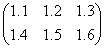定义成数组，名R

[5.2] 设数组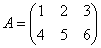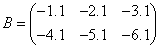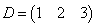E数组描述为(1:2,1:3)F数组描述为(1:3)，问下列数组表达式是否合法，不合法的说明理由，合法的写出计算结果：

(1) E=A+B

(2) E=ABS(B)+2

(3) E=B+C

(4) C=A+C

(5) F=D*D

(6) F=A(1:2,1)+B(1:3,1)

(7) F=A(1,1:3)+B(1,1:3)

[5.3] WHERE构造，使上题中A元素≤2时，置B相应元素为自身的绝对值，否则使B中元素加1，写出最后B的内容。

[5.4] 读入一个二维数组A，形状为(1:5,1:3)，任意输入具体数据。

(1) DO循环求全部数组元素和，再用数组内部专用求和函数求和，比较两者结果。

(2) DO循环求数组元素连乘积，再用数组内部求连乘积函数求整个数组元素之积，比较两者结果。

[5.5] 用动态数组存放任意读入的N个储款数，统计这次存款总数，而后释放数组，再把它存贮任意读入的M个取款数，统计共被取出的款额，求收支相抵的余额。